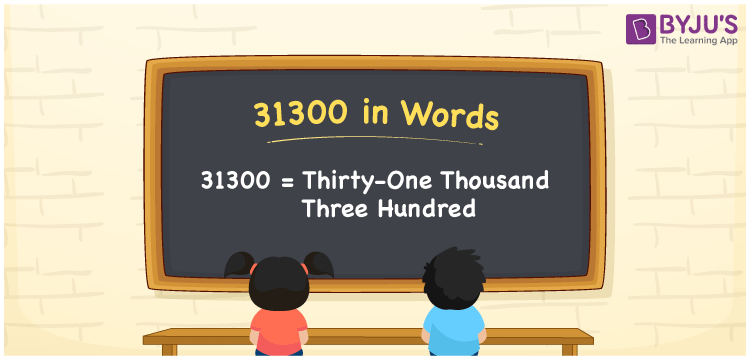# 31300 in Words

31300 in words can be written as Thirty-One Thousand Three Hundred. Suppose if you paint your house for Rs. 31300, then you can say that “I painted my house for Thirty-One Thousand Three Hundred Rupees”. In this article, you will understand the place value of 31300 with its conversion in words. Hence, 31300 can be read as “Thirty-One Thousand Three Hundred” in words.

 31300 in words Thirty-One Thousand Three Hundred Thirty-One Thousand Three Hundred in Numbers 31300

## 31300 in English Words## How to Write 31300 in Words?

Let us curate the place value chart of 31300 upon which numbers can be written in English words.

 Ten Thousands Thousands Hundreds Tens Ones 3 1 3 0 0

The expanded form of 31300 is provided below:

3 x Ten Thousand + 1 x Thousand + 3 x Hundred + 0 × Ten + 0 × One

= 3 x 10000 + 1x 1000 + 3 x 100 + 0 x 10 + 0 x 1

= 30000 + 1000 + 300

= 31300

= Thirty-One Thousand Three Hundred

Therefore, 31300 in words is written as Thirty-One Thousand Three Hundred.

31300 is a natural number that precedes 31301 and succeeds 31299.

31300 in words – Thirty-One Thousand Three Hundred

Is 31300 an odd number? – No

Is 31300 an even number? – Yes

Is 31300 a perfect square number? – No

Is 31300 a perfect cube number? – No

Is 31300 a prime number? – No

Is 31300 a composite number? – Yes

## Frequently Asked Questions on 31300 in Words

Q1

### How do you write the number 31300 in words?

31300 in words is Thirty-One Thousand Three Hundred.
Q2

### Find the value of 32000 minus 700.

The value of 32000 plus 700 is 31300. Therefore, 31300 in words is Thirty-One Thousand Three Hundred.
Q3

### Is 31300 a perfect square?

No, 31300 is not a perfect square as it cannot be expressed as the product of two integers which are equal.